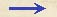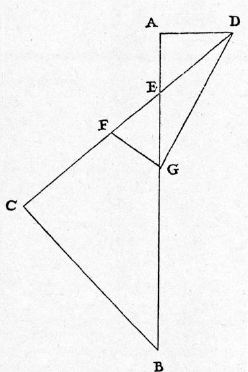Discorsi Propositions 2/16-th-13 Discorsi Proposition2/16-th-13THEOREMA XIII, PROPOSITIO XVI. THEOREM XIII, PROPOSITION XVISi plani inclinati et perpendiculi partes, quarum tempora lationum ex quiete sint aequalia, ad idem punctum componantur, mobile {20} veniens ex qualibet altitudine sublimiori, citius absolvet eandem partem plani inclinati, quam ipsam partem perpendiculi. If a limited inclined plane and a limited vertical line are drawn from the same point, and if the time required for a body, starting from rest, to traverse each of these is the same, then a body falling from any higher altitude will traverse the inclined plane in less time than is required for the vertical line. Sit perpendiculum EB et planum inclinatum CE, ad idem punctum E composita, quorum tempora lationum ex quiete in E sint aequalia; et in perpendiculo extenso sumptum sit quodlibet punctum sublime A, ex quo {30} demittantur mobilia: dico, tempore breviori absolvi planum inclinatum EC, quam perpendiculum EB post casum AE. Iungatur CB, et ducta horizontali AD, extendatur CE, illi occurrens in D, et CD, DE media {234} proportionalis sit DF, ipsarum vero BA, AE media sit AG, et ducantur FG, DG: et quia tempora lationum per EC, EB ex quiete in E sunt aequalia, erit angulus C rectus, ex corollario secundo propositionis sextae; estque rectus A, et anguli ad verticem E aequales; triangula igitur AED, CEB sunt aequiangula, et latera circa aequales angulos proportionalia; ergo ut BE ad EC, ita DE ad EA. Rectangulum ergo BEA est aequale rectangulo CED; et quia rectangulum CDE superat rectangulum CED quadrato ED, rectangulum vero BAE superat rectangulum BEA quadrato EA, excessus rectanguli CDE super rectangulo BAE, hoc est quadrati FD super quadrato AG, erit idem cum excessu quadrati DE super quadrato AE, qui excessus est {10} quadratum DA. Est igitur quadratum FD aequale duobus quadratis GA, AD, quibus est quoque aequale quadratum GD; ergo linea DF ipsi DG est aequalis, et angulus DGF aequalis angulo DFG, et angulus EGF minor angulo EFG, et latus oppositum EF minus latere EG. Modo si intelligamus, tempus casus per AE esse ut AE, erit tempus per DE ut DE; cumque AG media sit inter BA, AE, erit AG tempus per totam AB, et reliqua EG erit tempus per reliquam EB ex quiete in A; et similiter concludetur, EF esse tempus per EC post descensum DE, seu post casum AE: demonstratum autem est, EF minorem esse quam EG: ergo patet propositum. Let EB be the vertical line and CE the inclined plane, both starting from the common point E, and both traversed in equal times by a body starting from rest at E; extend the vertical line upwards to any point A, from which falling bodies are allowed to start. Then, I say that, after the fall through AE, the inclined plane EC will be traversed in less time than the perpendicular EB. Join CB, draw the horizontal AD, and prolong CE backwards until it meets the latter in D; let DF be a mean proportional between CD and DE while AG is made a mean proportional between BA and AE. Draw FG and DG; then {234} since the times of descent along EC and EB, starting from rest at E, are equal, (Condition 2/06-th-06-cor2) it follows, according to Corollary II of Proposition VI that the angle at C is a right angle; but the angle at A is also a right angle and the angles at the vertex E are equal; hence the triangles AED and CEB are equiangular and the sides about the equal angles are proportional; hence BE:EC = DE:EA. Consequently the rectangle BE.EA is equal to the rectangle CE.ED; and since the rectangle CD.DE exceeds the rectangle CE.ED by the square of ED, and since the rectangle BA.AE exceeds the rectangle BE.EA by the square of EA, it follows that the excess of the rectangle CD.DE over the rectangle BA.AE, or what is the same thing, the excess of the square of FD over the square of AG, will be equal to the excess of the square of DE over the square of AE, which excess is equal to the square of AD. Therefore FD^2 = GA^2 + AD^2 = GD^2. Hence DF is equal to DG, and the angle DGF is equal to the angle DFG while the angle EGF is less than the angle EFG, and the opposite side EF is less than the opposite side EG. If now we agree to represent the time of fall through AE by the length AE, (Condition 2/03-th-03-cor) then the time along DE will be represented by DE. And since AG is a mean proportional between BA and AE, (Condition 2/02-th-02-cor2) it follows that AG will represent the time of fall through the total distance AB, (Condition 2/11-th-11) and the difference EG will represent the time of fall, from rest at A, through the difference of path EB. (Condition 2/11-th-11) In like manner EF represents the time of descent along EC, starting from rest at D or falling from rest at A. But it has been shown that EF is less than EG; hence follows the theorem.Discorsi Propositions 2/16-th-13 Discorsi Proposition2/16-th-13